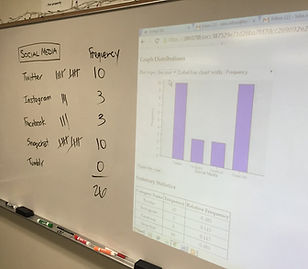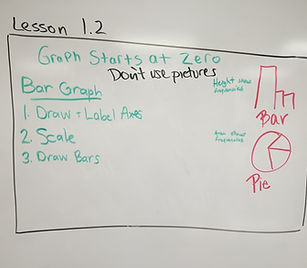## Chapter 1 - Day 4 - Lesson 1.2

##### Learning Targets
• Make and interpret bar charts of categorical data.

• Interpret bar charts.

• Identify what makes some graphs of categorical data deceptive.

##### Homework

We decided that we are going to give less homework problems with the hopes that students recognize the value of homework and work on it authentically.  The magic number we settled on is 5.  Only 5 homework problems.  Typically the first 2 or 3 will directly address the learning targets, the next 2 will be problems where they need to put it all together, and then 1 review problem (read Make It Stick if you want to know why – interleaving learning).  This means that at the start of each day, we often will only go over 1 homework problem.

##### Activity: What is Your Social Media of Choice?

We are going to try to have some sort of activity to start each new lesson.  We use the word “Activity” loosely, but as a general rule means that students are doing something within a real context.  Today, we asked “What is the most used social media app by students?”  Students collected the data for the class and displayed it in a frequency table and a relative frequency table (so we can connect new learning to previous learning).  Then we used iPads to make bar charts and pie graphs. We are going to use the applets found here for several activities in Chapter 1.